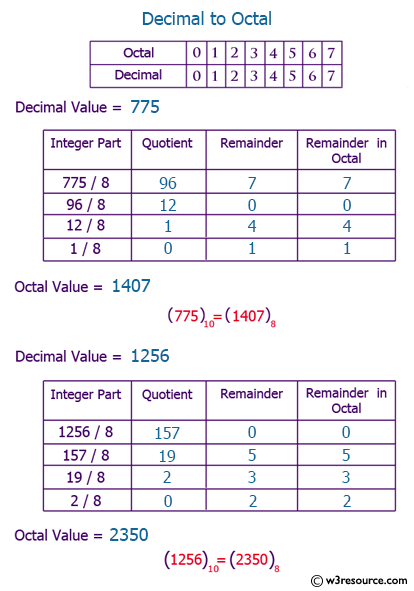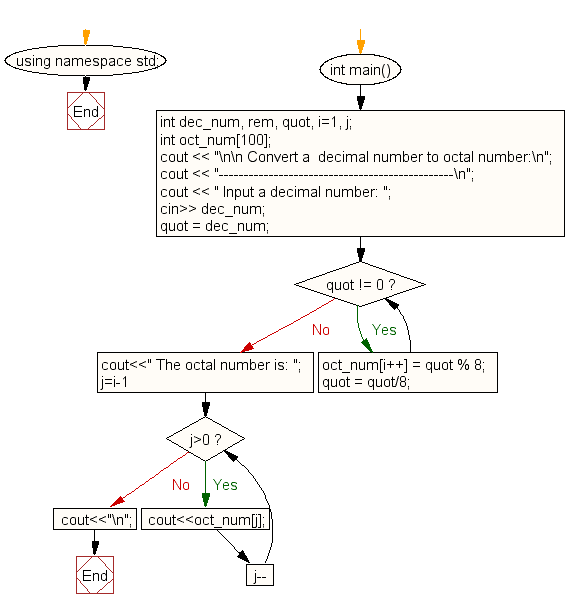﻿ C++ Exercises: Convert a decimal number to octal number - w3resource# C++ Exercises: Convert a decimal number to octal number

## C++ For Loop: Exercise-72 with Solution

Write a program in C++ to convert a decimal number to octal number.

Pictorial Presentation:Sample Solution:-

C++ Code :

``````#include <iostream>
#include <math.h>
using namespace std;

int main()
{
int dec_num, rem, quot, i=1, j;
int oct_num;
cout << "\n\n Convert a  decimal number to octal number:\n";
cout << "-----------------------------------------------\n";
cout << " Input a decimal number: ";
cin>> dec_num;
quot = dec_num;
while(quot != 0)
{
oct_num[i++] = quot % 8;
quot = quot/8;
}

cout<<" The octal number is: ";
for(j=i-1; j>0; j--)
{
cout<<oct_num[j];
}
cout<<"\n";
}
``````

Sample Output:

``` Convert a  decimal number to octal number:
-----------------------------------------------
Input a decimal number: 15
The octal number is: 17
```

Flowchart:C++ Code Editor:

Contribute your code and comments through Disqus.

What is the difficulty level of this exercise?

﻿